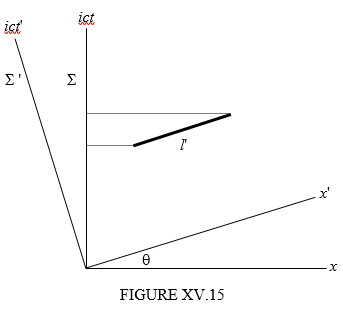$$\require{cancel}$$

# 15.13: Simultaneity

•• Contributed by Jeremy Tatum
• Emeritus Professor (Physics & Astronomy) at University of Victoria

If the time interval referred to one reference frame can be different when referred to another reference frame (and since time interval is merely one component of a four-vector, the magnitude of the component surely depends on the orientation in four space of the four axes) this raises the possibility that there might be a time interval of zero relative to one frame (i.e. two events are simultaneous) but are not simultaneous relative to another. This is indeed the case, provided that the two events do not occur in the same place as well as at the same time. Look at Figure XV.15.I have drawn two reference frames at an (imaginary) angle $$\theta$$ to each other. Think of $$\sum$$ as the railway station and of $$\sum'$$ as the railway train, and that the speed of the railway train is $$c\tan\theta$$ (You may have to go back to Section 15.3 or 15.7 to recall the relation of $$\theta$$ to the speed.) The thick line represents the interval between two events that are simultaneous when referred to $$\sum'$$, but are separated in space (one occurs near the front of the train; the other occurs near the rear). (Note also in this text that I am using the phrase “time interval” to denote the time-component of the “interval”. For two simultaneous events, the time interval is zero, and the interval is then merely the distance between the two events.)

While the thick line has zero component along the $$ict'$$ axis, its component along the $$ict$$ axis is $$l'\sin\theta$$. That is, $$ic(t_{2}-t_{1})=l'\sin\theta=l'\times i\beta\gamma$$.

Hence:

$t_{2}-t_{1}=\frac{\beta\gamma l'}{c}. \label{15.13.1}$

For example, if the events took place simultaneously 100,000 km apart in the train (it is a long train) and if the train were travelling at 95% of the speed of light ($$\gamma$$ = 3.203; it is a fast train), the two events would be separated when referred to the railway station by 1.01 seconds. The event near the rear of the train occurred first.